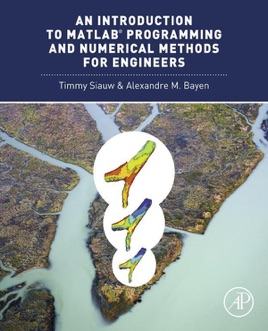• USD 92.99

## Descripción de editorial

Assuming no prior background in linear algebra or real analysis, An Introduction to MATLAB® Programming and Numerical Methods for Engineers enables you to develop good computational problem solving techniques through the use of numerical methods and the MATLAB® programming environment. Part One introduces fundamental programming concepts, using simple examples to put new concepts quickly into practice. Part Two covers the fundamentals of algorithms and numerical analysis at a level allowing you to quickly apply results in practical settings.

Tips, warnings, and "try this" features within each chapter help the reader develop good programming practicesChapter summaries, key terms, and functions and operators lists at the end of each chapter allow for quick access to important informationAt least three different types of end of chapter exercises — thinking, writing, and coding — let you assess your understanding and practice what you've learned